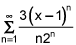Bob M.

# The power series  has a radius of convergence R = 2. Determine the interval of convergence.

The power serieshas a radius of convergence R = 2. Determine the interval of convergence.

 (-1, 3) [-1, 3] (-1, 3] [-1, 3)